# QlikView App Dev

Discussion Board for collaboration related to QlikView App Development.

cancel
Showing results for
Did you mean: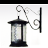Creator III

## Range sum issue

Hello Qlik developers,

I'm trying to create the no's below in red using the below expressions but my no's are not giving me the below output in red. I have written the same expressions for one of the table which is giving me the expected results. Can someone help me on this please?

Final: if(column(1) >0 and (isnull(fabs(above(column(1)))) or isnull(fabs(below(column(1))))), Column(1))

Base: IF((isnull(Above([VALUE])) OR ISNULL(BELOW([VALUE]))),NULL(),RangeSum (Above([VALUE],0,9)))

 Group Sub Group Value Increase Decrease Final Base Test A 75 75 Test B -10 10 65 Test C -15 15 50 Test D 5 5 55 Test E -8 8 47 Test1 F 4 4 51 Test1 G 6 6 57 Test1 H -13 13 44 Test1 ZZZ 74 74

Thanks

1 Solution

Accepted SolutionsMVP

hi

Try like below

IF((isnull(Above(Total [Value])) OR ISNULL(BELOW(Total [Value]))),NULL(),RangeSum (Above(Total [Value],0,9)))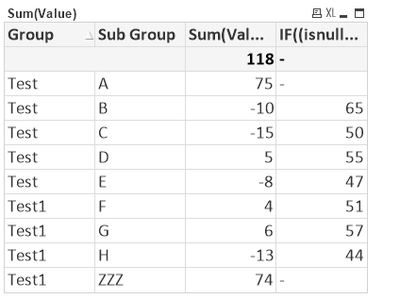Thanks & Regards,
Mayil Vahanan R
6 Replies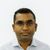MVP

Try this,

``````tab1:
LOAD *, If(Match([Sub Group],'A','ZZZ'), Value) As Final,
If(Not Match([Sub Group],'A','ZZZ'), K1) As Base;
Group, Sub Group, Value
Test, A, 75
Test, B, -10
Test, C, -15
Test, D, 5
Test, E, -8
Test1, F, 4
Test1, G, 6
Test1, H, -13
Test1, ZZZ, 74
];

Drop Field K1;``````MVP

Output: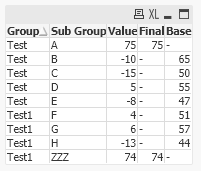Creator III
Author

Hi Saran,

Thank You for the response. I'm looking to achieve this on UI expression as my dimension values changes.

Thanks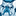Contributor

I have also same error but can't find solution please reply if anyone know.

MyAARPMedicareMVP

hi

Try like below

IF((isnull(Above(Total [Value])) OR ISNULL(BELOW(Total [Value]))),NULL(),RangeSum (Above(Total [Value],0,9)))Thanks & Regards,
Mayil Vahanan RCreator III
Author

Hi MayiVahanan,

The expression worked. Thanks a lot. 😊

ThanksCommunity Browser